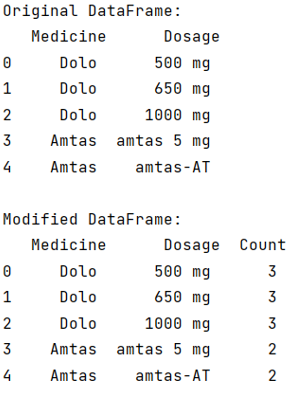# Create column of value_counts in Pandas dataframe

Given a DataFrame, we need to create a column called count which consist the value_count of the corresponding column value. By Pranit Sharma Last updated : September 18, 2023

Pandas is a special tool that allows us to perform complex manipulations of data effectively and efficiently. Inside pandas, we mostly deal with a dataset in the form of DataFrame. DataFrames are 2-dimensional data structures in pandas. DataFrames consist of rows, columns, and data.

## Problem statement

Given a DataFrame, we need to create a column called count which consist the value_count of the corresponding column value.

## Creating column of value_counts in Pandas dataframe

To achieve this task pandas provide us groupby() method which has an attribute called count. The Pandas's groupby() method counts first groups all the same values and then count attribute will returns an integer value which represents the count of these grouped values i.e., the occurrences of these column values.

Let us understand with the help of an example,

## Python program to create column of value_counts in Pandas dataframe

```# Importing pandas package
import pandas as pd

# Creating a Dictionary
d = {
'Medicine':['Dolo','Dolo','Dolo','Amtas','Amtas'],
'Dosage':['500 mg','650 mg','1000 mg','amtas 5 mg','amtas-AT']
}

# Creating a DataFrame
df = pd.DataFrame(d)

# Display original DataFrame
print("Original DataFrame:\n",df,"\n")

# Using groupby count
df['Count'] = df.groupby(['Medicine'])['Dosage'].transform('count')

# Display modified DataFrame
print("Modified DataFrame:\n",df)
```

### Output

The output of the above program is: